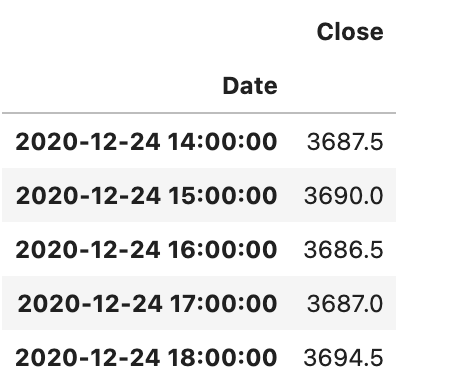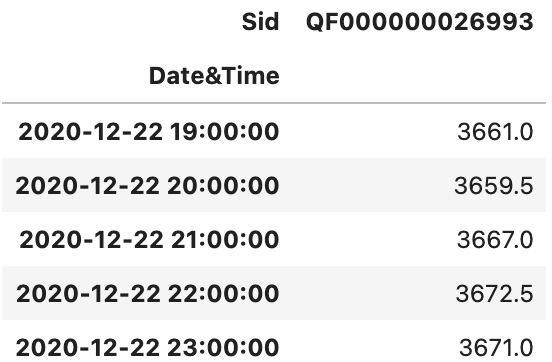Preface, if there is a better way to solve 1 & 2 in a Moonshot backtest, I’m not tied to either attempt below.

I need 2 pieces within Moonshot Futures:

1. Rolls are stitched together (which `download_history_file` does nicely).
2. The index is `DatetimeIndex`, not a `tz-aware` index that `download_history_file` gives.

Doing the below I get a resultant df:

``````def prices_to_signals(self, prices):

filepath_or_buffer="es-1hr.csv",
fields=["Close"],
cont_fut="concat")

df_es["Date"] = pd.to_datetime(df_es['Date'],
infer_datetime_format=True,
utc=True).dt.strftime('%Y-%m-%d %H:%M:%S')

# redefine prices
prices = df_es[["Date", "Close"]]
prices.index = prices["Date"]
prices.index = pd.to_datetime(prices.index)
closes = prices.drop(["Date"], axis=1)
``````This effectively solves 1 & 2 but requires quite a bit of manipulation to download a csv file then re-upload. And it requires all future prices dataframes to need it, as in `positions_to_gross_returns`. Then the `backtest` functionality throws errors.

Alternatively, if using the prices df directly you can satisfy the `DatetimeIndex` requirement but not the stitching (without manually coding which is what `cont_fut='concat'` is useful for).

from quantrocket import get_prices
prices = get_prices(“es-1hr”, fields=[“Close”], start_date=‘2020-01-01’, end_date=‘2020-12-24’)
prices = prices.loc[“Close”]
prices = prices.reset_index(level=[0,1])
prices[[“Date”, “Time”]] = prices[[“Date”, “Time”]].astype(str)
prices.index = pd.to_datetime(prices[‘Date’] + " " + prices[‘Time’])
prices = prices.drop([“Date”, “Time”], axis=1)

Check out the `CONT_FUT` parameter on Moonshot:

https://www.quantrocket.com/docs/api/#moonshot.Moonshot

`CONT_FUT='concat'` did the trick.
For anyone else, I had to make a few adjustments to have a specific `DateTimeindex` rather than a `MultiIndex`:

``````class MyClassName(Moonshot):

CODE = "my-strategy"
DB = "es-1hr"
FIELDS=["Close"]
CONT_FUT='concat'

def prices_to_signals(self, prices):

closes = prices.loc["Close"]
closes = closes.reset_index()

closes['Date&Time'] = pd.to_datetime(closes['Date'].astype(str) + '/' + closes['Time'].astype(str))
closes = closes.set_index('Date&Time').drop(['Date','Time'], axis=1)
``````

`closes.tail()`